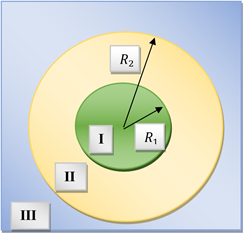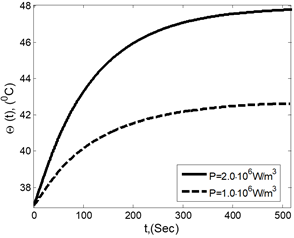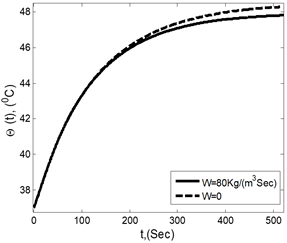# Heat Exchange Within the Surrounding Biological Tissue During Magnetic Hyperthermia

Heat Exchange Within the Surrounding Biological Tissue During Magnetic Hyperthermia
Theoretical and Mathematical Physics Department, Institute of Natural Sciences and Mathematics, Ural Federal University, Lenin Ave, 51, Ekaterinburg 620083, Russia

Mathematics and Computer Science Department, Faculty of Science, Menoufia University, Shebin El-Kom 32511, Egypt

M.N. Mikheev Institute of Metal Physics of the Ural Branch of the Russian Academy of Sciences, Ekaterinburg 620083, Russia

Corresponding Author Email:
alibakrm@yahoo.com
Page:
196-200
|
DOI:
https://doi.org/10.18280/mmep.070204
10 December 2019
|
Accepted:
13 May 2020
|
Published:
30 June 2020
| Citation

OPEN ACCESS

Abstract:

The paper deals with mathematical modeling and theoretical study of the heat distribution within the surrounding biological tissue during the effect of the magnetic hyperthermia. The mathematical model is formulated and solved numerically by using the finite difference method. The intensity of heat production is used in the present model. The obtained results allow predicting the temperature change in tumor as well as in the surrounding tissue depending on intensity of the tumor heating.

Keywords:

bioheat transfer equation, mathematical modeling, biological tissue, hyperthermia

1. Introduction

Magnetic hyperthermia is a new type of cancer treatment [1-4], allowing to achieve selective heating of a tumor without damaging of the surrounding healthy tissues. The main idea of this method is in embedding of magnetic nanoparticles in a tumor region and heating them with the help of alternating magnetic field. In the temperature corridor 42-50℃ the tumor cells undergo protein destruction, which leads to the cells death, whereas the cells of the healthy tissue, being more temperature resistant, are not injured. Magnetic hyperthermia is a way of tissue heating, by using thermal effect produced by embedded magnetic nanoparticles under the action of oscillating or rotating magnetic field.

In physical models of magnetic hyperthermia, biological media are usually considered as a blood-flooded matrix composed of cells and interstitial space [5-9]. The tumor models with a well-defined geometry, typically spherical, are added to this simple picture so that they remain surrounded by a finite or infinite layer of healthy tissue with infinity radius. Specific heat, thermal conductivities of the involved biological media - tumors, viscera, muscle, fat, skin, etc., must be included in the model.

The development of mathematical models of heat transfer in living tissues has been a topic of interest for various biologists, physicians, mathematicians and engineers. The accurate explanation of the thermal interaction between vasculature and tissues is necessary for the development of medical technology of tumor diseases [10, 11]. Thermal models of bioheat transfer equations in living tissue and thermal dose equivalence due to hyperthermia are presented in [12, 13]. These works focus both on the basic formulations of the bioheat transfer equations in the living tissue and on the determination of thermal dose during thermal therapy. The distributions of temperature inside the heated tissues, generally controlled by heating modalities, are got by solving the bioheat transfer equation. A model for heating magnetic fluid with the help of alternating magnetic field is presented in . The heat sources, i.e. magnetic nanoparticles, must be included to complete the basic tumor model of magnetic hyperthermia. These sources are determined by the particles size distribution, their magnetic properties, spatial distribution, which determines the formation of “hot spots” and the uniformity of heat deposition in the tissue where the magnetic nanoparticles are infused [15-20]. However, this model is difficult to be used in practical applications, since the obtained analytical solutions are too complicated and cumbersome. Some analytical solution of the system of dimensional Fourier and non-Fourier bioheat transfer equations in skin tissue exposed to an instantaneous heating condition is presented by Askarizadeh and Ahmadikia .

In this work, we present a theoretical study and mathematical modeling of a tumor heating taking into account the heat exchange with the surrounding biological tissue. Numerical techniques are used to solve the equations of the mathematical model with heat source of the permanent intensity, located in a spherical region, which models the tumor. Some discussion of important parameters research issues in hyperthermia problem are also addressed.

2. Formulation of the Problem

The tumor is loaded with magnetic nanoparticle, while the healthy tissue, surrounding this tumor, does not contain the particles. Thus only the tumor is directly heated by the applied field. We suppose that the perfusion term, corresponding to the heat transfer into the blood, is proportional to the volumetric blood ﬂow and to the difference between temperature of the local tissue and the arterial temperature. The blood perfusion is homogeneous throughout the healthy and affected tissue, since blood capillaries are typically more or less homogeneously distributed in the tissue bed. We study magnetic hyperthermia in a spherical tumor tissue. The illustration of the model is shown in Figure 1.

## 1.pngFigure 1. Illustration of the model of the heated tumor region (I) separated from the main part (III) of biological tissue with transition region (II)

Let us denote radius of the spherical tumor region as R1; the average specific heat capacity and the thermal conductivity of this area as с1, λ1 respectively; the intensity of heat generation, under an alternative magnetic filed, per unit volume of the tumor as P.

We will take into account that the tumor region can be separated from the environment by an area with a modified structure, therefore with a modified thermo-physical characteristic. In Figure 1, this transition layer is illustrated by the area II with the internal and external radiuses R1 and R2 respectively. Specific heat capacity and thermal conductivity of this region will be denoted as с2 and λ2. The main part III of the biological tissue is outside this transition region; in this region the heat can be carried away by the bloodstream. Thermal properties of the biological tissue are denoted by с3 and λ3. ρi is the density in region i. This work aims to develop a new approach toward solving the bio-heat transfer equations for different regions of biological system subjected to hyperthermia effect which depends on the magnetic nanoparticles that are injected into the tumor region and distributed in the tumor cells and It is considered to the heat source of power dissipation.

The mathematical model of the heat distribution in the zone of hyperthermia can be presented as:

At r<R1:

$c_{1} \rho_{1} \frac{\partial \Theta_{1}}{\partial t}=\lambda_{1} \frac{1}{r^{2}} \frac{\partial}{\partial r}\left(r^{2} \frac{\partial \Theta_{1}}{\partial r}\right)+P$     (1)

At R1<r<R2:

$c_{2} \rho_{2} \frac{\partial \Theta_{2}}{\partial t}=\lambda_{2} \frac{1}{r^{2}} \frac{\partial}{\partial r}\left(r^{2} \frac{\partial \Theta_{2}}{\partial r}\right)$

At r>R2:

$c_{3} \rho_{3} \frac{\partial \Theta_{3}}{\partial t}=\lambda_{3} \frac{1}{r^{2}} \frac{\partial}{\partial r}\left(r^{2} \frac{\partial \Theta_{3}}{\partial r}\right)-W c_{w} \Theta_{3}$

Here r is the distance from the tumor center; Θ1(r,t), Θ2(r,t), Θ3(r,t) are the differences of temperature in the respective tissue areas and the temperature at an infinite distance from the tumor; W and cw are the empirical parameters describing the thermal effects due to the blood circulation. The last term in (1) effectively describes the heat carried away by the circulation of blood from the tissue adjacent to the tumor region .

We estimate the intensity P of the heat production, by using results  of theoretical study of the heat production in a system of ferromagnetic particles placed in an alternating magnetic field, taking into account magnetic interaction between these particles. The Neel mechanism of the particles remagnetization  was supposed. Magnetic interaction between them has been estimated in the frames of the mathematically regular approximation of pair interaction. The result reads:

\begin{aligned} \mathrm{P}=\pi \phi \mu_{0} \mathrm{H}_{0} \mathrm{M}_{\mathrm{p}} \alpha_{0} & \frac{\omega \tau}{1+(\omega \tau)^{2}}\left(1+\frac{1}{16} \alpha_{0}^{2}\left(\frac{2(\omega \tau)^{2}-3}{1+(\omega \tau)^{2}}\right)\right.\\ &+\frac{8 \phi \gamma}{1+(\omega \tau)^{2}}[2\\ &+\alpha_{0}^{2}\left[\frac{(3+\omega \tau)\left(10(\omega \tau)^{2}-7\right)}{16 \omega \tau\left(1+(\omega \tau)^{2}\right)}\right.\\ &+\frac{1}{144}\left(2(\omega \tau)^{2}\right.\\ &-1)\left(12+\frac{5(\omega \tau)^{2}-1}{\omega \tau\left(1+9(\omega \tau)^{2}\right)}\right.\\ &\left.\left.\left.\left.+\frac{9\left(2(\omega \tau)^{2}-3\right)}{\left(1+(\omega \tau)^{2}\right)\left(2(\omega \tau)^{2}-1\right)}\right)\right]\right]\right) \end{aligned}     (2)

Here, $\gamma=\frac{\mu_{0} \mathrm{m}^{2}}{4 \pi(2 a)^{3} \mathrm{k}_{\mathrm{B}} \mathrm{T}}$ is the dimensionless parameter of the dipolar interaction energy between two particles to the thermal energy $\mathrm{k}_{\mathrm{B}} \mathrm{T}, \mathrm{m}=\mathrm{M}_{\mathrm{p} \mathrm{V}}, \mathrm{v}=\frac{4}{3} \pi a^{3}$ is the volume of particle, $a$ is the radius particle, $\alpha_{0}=\frac{\mu_{0} \mathrm{mH}_{0}}{\mathrm{k}_{\mathrm{B}} \mathrm{T}}$ is the dimensionless of magnetic field. $\mu_{0}=4 \pi \times 10^{-7} \frac{\mathrm{T} \mathrm{m}}{\mathrm{A}} ;$ is magnetic permeability of free space, $k_{B}=1.38 \cdot 10^{-23} \frac{J}{0_{K}} ;$ is Boltzmann constant $\mathrm{T}=300^{\circ} \mathrm{K} ;$ is absolute temperature. The parameters $\mathrm{H}_{0}, \ldots$ are given in Table 1 in the section of discussion and results.

For following Figure 1, the temperature distributions for each region of biological tissue are subject to initial and boundary conditions and use that, to solve the system Eqns. (1, 2), then the symmetry and boundary conditions can be expressed as:

$\frac{\partial \Theta(r, t)}{\partial r}=0 ; r=0$     (3a)

$\Theta_{1}=\Theta_{2} ; \lambda_{1} \frac{\partial \Theta_{1}}{\partial r}=\lambda_{2} \frac{\partial \Theta_{2}}{\partial r}, r=R_{1}:$

$\Theta_{2}=\Theta_{3} ; \lambda_{2} \frac{\partial \Theta_{2}}{\partial r}=\lambda_{3} \frac{\partial \Theta_{3}}{\partial r}, r=R_{2}$

$\Theta_{3} \rightarrow 0, r \rightarrow \infty$

And initial conditions are

$t=0, \Theta_{1}=\Theta_{2}=\Theta_{3}=37^{0} \mathrm{C}$     (3b)

3. Numerical Technique

On the basis of the reduced system of Eqns. (1-2) and the finite difference method, we will use the following substitutions:

$\frac{\partial \Theta}{\partial t} \rightarrow \frac{\Theta_{i}^{N}-\Theta_{i}}{\Delta t}$

where, $\Theta_{i}^{N}$ is the temperature at current i-time step in the region $\mathrm{N},$ whereas $\Theta_{\mathrm{i}}$ is the temperature in the previous step. The index i marks the coordinate of the $\mathrm{r}_{\mathrm{i}}, \triangle \mathrm{t}$ is the time step. The spatial derivatives of Eqns. $(1-3)$ are replaced by using central differencing approximation:

$\frac{\partial \Theta}{\partial \mathbf{r}}=\frac{\Theta_{i+1}-\Theta_{i-1}}{2 \Delta \mathbf{r}}$ and $\frac{\partial^{2} \Theta}{\partial r^{2}}=\frac{\Theta_{i+1}-2 \Theta_{i}+\Theta_{i-1}}{(\Delta r)^{2}}$

where, $\mathrm{r}_{i}=\mathrm{i} \triangle \mathrm{r} ; \mathrm{i}=0,1,2, \ldots, \mathrm{n}+1$, the coordinate in increments of $\Delta \mathbf{r}=\frac{1}{n+1}$. We take the system of heat distribution of the present model in numerical techniques that can be expressed as:

Region I:

${\Theta_{\mathrm{i}}^{\mathrm{N}}=\Theta_{\mathrm{i}}+\frac{\lambda_{1}}{\mathrm{c}_{1}} \frac{\Delta \mathrm{t}}{\rho_{\mathrm{i}}(\triangle \mathrm{r})^{2}}\left(\left(1+\frac{\Delta \mathrm{r}}{\mathrm{r}_{\mathrm{i}}}\right) \Theta_{\mathrm{i}+1}-2 \Theta_{\mathrm{i}}\right.}{\left.+\left(1-\frac{\Delta \mathrm{r}}{\mathrm{r}_{\mathrm{i}}}\right) \Theta_{\mathrm{i}-1}\right)+\frac{\Delta \mathrm{t}}{\mathrm{c}_{1}} \mathrm{P}}$     (4a)

Region II:

\begin{aligned} \Theta_{\mathrm{i}}^{\mathrm{N}}=\Theta_{\mathrm{i}}+\frac{\lambda_{2}}{\mathrm{c}_{2}} \frac{\Delta \mathrm{t}}{\rho_{2}(\Delta \mathrm{r})^{2}}\left(\left(1+\frac{\Delta \mathrm{r}}{\mathrm{r}_{\mathrm{i}}}\right)\right.& \Theta_{\mathrm{i}+1}-2 \Theta_{\mathrm{i}} \\\left.+\left(1-\frac{\Delta \mathrm{r}}{\mathrm{r}_{\mathrm{i}}}\right) \Theta_{\mathrm{i}-1}\right) \end{aligned}    (4b)

Region III:

\begin{aligned} \Theta_{i}^{N}=\Theta_{i}+\frac{\lambda_{3}}{c_{3}} \frac{\Delta t}{\rho_{3}(\Delta r)^{2}}\left(\left(1+\frac{\Delta r}{r_{i}}\right)\right.& \Theta_{i+1}-2 \Theta_{i} \\ &\left.+\left(1-\frac{\Delta r}{r_{i}}\right) \Theta_{i-1}\right)-\frac{W c_{w} \Delta t}{c_{3}} \Theta_{i} \end{aligned}     (4c)

And also, the relations (3) are transformed to new relations as:

Initial conditions:

$t=0, \Theta_{1}=\Theta_{2}=\Theta_{3}=37^{\circ} \mathrm{C}$     (5a)

Boundary conditions:

1- At r=0:

$\Theta^{N}(0)=\Theta^{N}(\Delta r)$     (5b)

2- At r=R1:

$\Theta_{\mathrm{R}_{1}}^{\mathrm{N}}=\frac{1}{\left(1+\frac{\lambda_{2}}{\lambda_{1}}\right)}\left(\Theta_{\mathrm{R}_{1-\Delta} \mathrm{r}}^{\mathrm{N}}+\frac{\lambda_{2}}{\lambda_{1}} \Theta_{\mathrm{R}_{1+\Delta \mathrm{r}}}^{\mathrm{N}}\right)$     (5c)

3- At r=R2:

$\Theta_{\mathrm{R}_{2}}^{\mathrm{N}}=\frac{1}{\left(1+\frac{\lambda_{3}}{\lambda_{2}}\right)}\left(\Theta_{\mathrm{R}_{2-\Delta \mathrm{r}}}^{\mathrm{N}}+\frac{\lambda_{3}}{\lambda_{2}} \Theta_{\mathrm{R}_{2+\Delta \mathrm{r}}}^{\mathrm{N}}\right)$     (5d)

4- At r→∞,

$\Theta_{i} \rightarrow 0, i=1,2,3$     (5e)

The system Eqns. (4-5) can be solved numerically to get the heat exchange of temperature surrounding the biological tissues.

4. Results and Discussions

To show the presented results, we calculate the intensity of heat production P by using Table 1 that given in the theoretical study of the heat production in a system of ferromagnetic particles  placed in an alternating magnetic field, taking into account magnetic interaction between these particles. The values of the physical parameters of the system, used for solution of the problem (1-3) are given Table 2.

Table 1. Values of parameters used for calculation of the intensity P heat of the heat production by using the results 

 Symbol Quantity Value H0 Magnetic field, ($\frac{K A}{m}$). 15.5 a Radius of particle, (nm). 11 ϕ Particle volume concentration, (%). 10 Mp Saturated magnetization, ($\frac{A}{m}$). 5.1×105 ω Angular frequency, ($\frac{1}{\operatorname{Sec}}$). 5.0×103 τ Time of the particle Néel remagentization, (Sec). 2×10-3

Table 2. The numerical values of physical parameters, used at the numerical calculations

 Parameter Value Unit λ1 0.5 W/(m 0C) λ2 0.45 W/(m 0C)) λ3 0.4 W/((m 0C)) ρi 1000 Kg/m3 R1 0.003 m R2 0.0045 m c1 4180 J/(m3 0C) c2 4000 J/(m3  0C) c3 3800 J/(m3  0C) cw 3344 J/(m3  0C) W 8 Kg/(m3∙Sec)

Figure 2 shows the results of numerical solution of the problem (1, 2). We note that the temperature distribute in the heated tumor region (I) separated from the main part (III) of biological tissue with transition region (II) where, Figure 2 reveals that profiles of temperature distribution is very close in the regions of therapeutic in biotissue regions and kept to the survive region when the temperature profile approach to zero. We remark there no exists significant penetration of the therapeutic temperatures to the outer region because thermal conductivity is small. Note that, the temperature profiles rend of the temperature curve, and addition to satisfies and justifies the boundary conditions (3) along horizental direction

## 2.pngFigure 2. Θ (r) as a function of the radial coordinate r for different values of time t after the start of heating of the tumor area; heat intensity $P=2 \cdot 10^{6} \frac{\mathrm{W}}{\mathrm{m}^{3}}$

## 3.pngFigure 3. The temperature Θ (0, t) in the center of the cell at different values of the intensity of the heat production P

## 4.pngFigure 4. The temperature Θ (0, t) in the center of the cell at different values of the intensity of the parameter, W

Figure 3 shows the time dependence of the temperature in the center of the heated region. Moreover, the temperature increases when the intensity of heat production (it is called magnetic hyperthermia) is increasing. The magnetic hyperthermia is depended on the physical parameters such as size of particles which control for the magnetic anisotropy and knowing the scale of hyperthermia that will use to treat the tumor cells in biological tissue.

Figure 4 displays effect of parameter W of the blood perfusion rate on the temperature $\Theta$ in the tumor center. As expected, this temperature decreases with W. These results allow predicting the temperature change in the tumor and in the surrounding tissue depending on the heating intensity and the tumor size. In general, our results are agreement with the given results [8, 22] where authors  solved the similar problem of bioheat equation by Laplace transforms.

5. Conclusion

We present the mathematical model and results of numerical calculations of temperature in the tumor region and in surrounding biological tissue at the addressed heating of the tumor. Intensity of the heat production in the tumor region is determined on the basis of the mathematically regular given model of the magneto-hyperthermia in a system of particles with the Neel mechanism of remagnetization. The heating dissipates within a relatively small distance from the center of the perfused tumor, which can be used advantageously to preserve the surrounding healthy tissue. This conclusion must be taken into account at the development of the technology of magnetic hyperthermia therapy of tumor diseases.

Acknowledgment

This paper has been supported by RFFI, grants 18-08-00178, 19-52-45001 and the state program of the Ministry of Science and Higher Education of the Russian Federation (theme “Magnet” and Contract No. 02.A03.21.006).

We thank the editor and anonymous referees for their constructive comments and recommendations.

References

 Liangruksa, M., Ganguly, R., Puri, I.K. (2011). Parametric investigation of heating due to magnetic fluid hyperthermia in a tumor with blood perfusion. Journal of Magnetism and Magnetic Materials, 323(6): 708-716. https://doi.org/10.1016/j.jmmm.2010.10.027

 Abu-Bakr, A.F., Zubarev, A.Y. (2019). Hyperthermia in a system of interacting ferromagnetic particles under rotating magnetic field. Journal of Magnetism and Magnetic Materials, 477: 404-407. https://doi.org/10.1016/j.jmmm.2018.07.010

 Attar, M.M., Haghpanahi, M., Amanpour, S., Mohaqeq, M. (2014). Analysis of bioheat transfer equation for hyperthermia cancer treatment. Journal of Mechanical Science and Technology, 28(2): 763-771. https://doi.org/10.1007/s12206-013-1141-4

 Abu-Bakr, A.F., Zubarev, A.Y. (2020). Effect of ferromagnetic nanoparticles aggregation on magnetic hyperthermia. The European Physical Journal Special Topics, 229(2-3): 323-329. https://doi.org/10.1140/epjst/e2019-900027-6

 Périgo, E.A., Hemery, G., Sandre, O., Ortega, D., Garaio, E., Plazaola, F., Teran, F.J. (2015). Fundamentals and advances in magnetic hyperthermia. Applied Physics Reviews, 2(4): 041302. https://doi.org/10.1063/1.4935688

 Kappiyoor, R., Liangruksa, M., Ganguly, R., Puri, I.K. (2010). The effects of magnetic nanoparticle properties on magnetic fluid hyperthermia. Journal of Applied Physics, 108(9): 094702. https://doi.org/10.1063/1.3500337

 Gupta, P.K., Singh, J., Rai, K.N., Rai, S.K. (2013). Solution of the heat transfer problem in tissues during hyperthermia by finite difference–decomposition method. Applied Mathematics and Computation, 219(12): 6882-6892. https://doi.org/10.1016/j.amc.2013.01.020

 Cheng, P.J., Liu, K.C. (2009). Numerical analysis of Bio-heat transfer in a spherical tissue. Journal of Applied Sciences, 9(5): 962-967. https://doi.org/10.3923/jas.2009.962.967

 Majchrzak, E., Turchan, Ł. (2017). Numerical analysis of tissue heating using the bioheat transfer porous model. Computer Assisted Methods in Engineering and Science, 20(2): 123-131. https://doi.org/10.2495/HT140411

 Gupta, P.K., Singh, J., Rai, K.N. (2013). A numerical study on heat transfer in tissues during hyperthermia. Mathematical and Computer Modeling, 57(5): 1018-1037. https://doi.org/10.1016/j.mcm.2011.12.050

 Javidi, M., Heydari, M., Karimi, A., Haghpanahi, M., Navidbakhsh, M., Razmkon, A. (2014). Evaluation of the effects of injection velocity and different gel concentrations on nanoparticles in hyperthermia therapy. Journal of biomedical physics & engineering, 4(4): 151. https://doi.org/10.1080/02656730801907937

 Shih, T.C., Kou, H.S., Liauh, C.T., Lin, W.L. (2002). Thermal models of bioheat transfer equations in living tissue and thermal dose equivalence due to hyperthermia. Biomedical Engineering: Applications, Basis and Communications, 14(2): 86-96. https://doi.org/10.4015/S1016237202000139

 Kengne, E., Lakhssassi, A. (2015). Bioheat transfer problem for one-dimensional spherical biological tissues. Mathematical Biosciences, 269: 1-9. https://doi.org/10.1016/j.mbs.2015.08.012

 Rosensweig, R.E. (2002). Heating magnetic ﬂuid with alternating magnetic ﬁeld. Journal of Magnetism and Magnetic Materials, 252: 370-374. https://doi.org/10.1016/S0304-8853(02)00706-0

 Dutz, S., Hergt, R. (2013). Magnetic nanoparticle heating and heat transfer on a microscale: basic principles, realities and physical limitations of hyperthermia for tumour therapy. International Journal of Hyperthermia, 29(8): 790-800. https://doi.org/10.3109/02656736.2013.82299

 Zubarev, A.Y., Iskakova, L.Y., Abu-Bakr, A.F. (2017). Magnetic hyperthermia in solid magnetic colloids. Physica A: Statistical Mechanics and its Applications, 467: 59-66. https://doi.org/10.1016/j.physa.2016.10.045

 Coïsson, M., Barrera, G., Celegato, F., Martino, L., Vinai, F., Martino, P., Tiberto, P. (2016). Specific absorption rate determination of magnetic nanoparticles through hyperthermia measurements in non-adiabatic conditions. Journal of Magnetism and Magnetic Materials, 415: 2-7. https://doi.org/10.1016/j.jmmm.2015.11.044

 Bagaria, H.G., Johnson, D.T. (2005). Transient solution to the bioheat equation and optimization for magnetic fluid hyperthermia treatment. International Journal of Hyperthermia, 21(1): 57-75. https://doi.org/10.1080/02656730410001726956

 Sawicki, B., Miaskowski, A. (2014). Nonlinear higher-order transient solver for magnetic fluid hyperthermia. Journal of Computational and Applied Mathematics, 270: 143-151. https://doi.org/10.1016/j.cam.2014.02.008

 Abu-Bakr, A.F., Zubarev, A. (2019). Effect of interparticle interaction on magnetic hyperthermia: homogeneous spatial distribution of the particles. Philosophical Transactions of the Royal Society A, 377(2143): 20180216. https://doi.org/10.1098/rsta.2018.0216

 Askarizadeh, H., Ahmadikia, H. (2015). Analytical study on the transient heating of a two-dimensional skin tissue using parabolic and hyperbolic bioheat transfer equations. Applied Mathematical Modelling, 39(13): 3704-3720. https://doi.org/10.1016/j.apm.2014.12.003

 Giordano, M.A., Gutierrez, G., Rinaldi, C. (2010). Fundamental solutions to the bioheat equation and their application to magnetic fluid hyperthermia. International Journal of Hyperthermia, 26(5): 475-484. https://doi.org/10.3109/02656731003749643